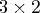Previous Page | Next Page

 Language Reference

## SHAPE Function

reshapes and repeats values

The inputs to the SHAPE function are as follows:
matrix
is a numeric or character matrix or literal.

nrow
gives the number of rows of the new matrix.

ncol
gives the number of columns of the new matrix.

is a fill value.
The SHAPE function shapes a new matrix from a matrix with different dimensions; nrow specifies the number of rows, and ncol specifies the number of columns in the new matrix. The operator works for both numeric and character operands. The three ways of using the function are outlined in the following list:

• If only nrow is specified, the number of columns is determined as the number of elements in the object matrix divided by nrow. The number of elements must be exactly divisible; otherwise, a conformability error is diagnosed.
• If both nrow and ncol are specified, but not pad-value, the result is obtained moving along the rows until the desired number of elements is obtained. The operation cycles back to the beginning of the object matrix to get more elements, if needed.
• If pad-value is specified, the operation moves the elements of the object matrix first and then fills in any extra positions in the result with the pad-value.
If nrow or ncol is specified as 0, the number of rows or columns, respectively, becomes the number of values divided by ncol or nrow.

For example, the following statement produces the result shown:

```
r=shape(12,3,4);
```

```
R             3 rows      4 cols    (numeric)

12        12        12        12
12        12        12        12
12        12        12        12
```

The following statement produces the result matrix by moving along the rows until the desired number of elements is obtained, cycling back as necessary:

```
r=shape(77,1,5);
```
Here is the output:
```
R        1 row       5 cols    (numeric)

77        77        77        77        77
```
The following statement has nrow specified and converts thematrix into amatrix:
```
r=shape({1 2, 3 4, 5 6},2);
```
Here is the output:
```

R             2 rows      3 cols    (numeric)

1         2         3
4         5         6
```
The following statement demonstrates the cycling back and repetition of elements in row-major order until the number of elements desired is obtained:
```
r=shape({99 31},3,3);
```
Here is the output:
```
R             3 rows      3 cols    (numeric)

99        31        99
31        99        31
99        31        99
```Previous Page | Next Page | Top of Page